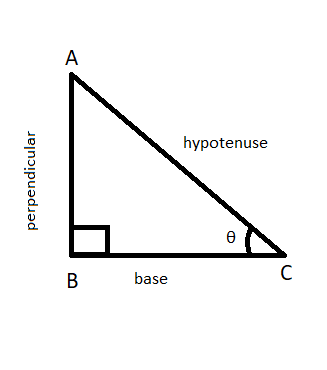GeeksforGeeks App
Open AppBrowser
Continue

# Evaluate sin 35° sin 55° – cos 35° cos 55°

Trigonometry is basically the study of the relationship between the angles and the sides of a triangle. It is one of the widely used topics of Mathematics that is used in daily life. t involves operations on a right-angled triangle i.e. a triangle having one of the angles equal to 90°. There are some terms that we should know before going further. These terms are,

1. Hypotenuse – it is the side opposite to the right angle in a right-angled triangle. It is the longest side of a right-angled triangle. In Figure 1, side AC is the hypotenuse.
2. Perpendicular – the perpendicular of a triangle, corresponding to a particularly acute angle θ is the side opposite to the angle θ. In Figure 1, side AB is the perpendicular corresponding to angle θ.
3. Base -it is the side adjacent to a particularly acute angle θ. In Figure 1 side BC is the base corresponding to angle θ.Figure 1

As earlier said, trigonometry depicts the relationship between the angles and sides of a right-angled triangle. These relationship is represented by standard ratios and are given as follows:

• Sine (sin) – the sine of an angle θ is the ratio of the length of the perpendicular, corresponding to the angle θ, to the length of the hypotenuse of the triangle.

sin θ = perpendicular/hypotenuse = p/h

• Cosine (cos) – the cosine of an angle θ is the ratio of the length of the base, corresponding to the angle θ, to the length of the hypotenuse of the triangle.

cos θ = base/hypotenuse = b/h

• Tangent (tan) – the tangent of an angle θ is the ratio of the length of the perpendicular, corresponding to the angle θ, to the length of the base for the particular angle of the triangle.

tan θ = perpendicular/base = p/b

• Cotangent (cot) – it is the reciprocal of a tangent.

cot θ = 1/tan θ = base/perpendicular = b/p

• Secant (sec) – it is the reciprocal of cosine.

sec θ = 1/cos θ = hypotenuse/base = h/b

• Cosecant (cosec) :- it is the reciprocal of sine.

cosec θ = 1/sin θ = hypotenuse/perpendicular = h/p

### Trigonometric functions of Complementary angles

One of the relations of trigonometry includes the concept of complementary angles. Complementary angles are a set of two angles, say x and y, such that on adding them they evaluate to 90°. Therefore, we can say x = 90° – y. There exist a special complementary relation between the trigonometric ratios as given below

Between sin and cos

sin(90° – x) = cos x

cos(90° – x) = sin x

Between tan and cot

tan(90° – x) = cot x

cot(90° – x) = tan x

Between sec and cosec

sec(90° – x) = cosec x

cosec(90° – x) = sec x

To solve the above expression we will need this complementary relationship between the trigonometric ratios.

### Evaluate sin 35° sin 55° – cos 35° cos 55°

Solution:

sin 35° sin 55° – cos 35° cos 55°

= sin (90° – 55°) sin 55° – cos (90° – 55°) cos 55°

= cos 55° sin 55° – sin 55° cos 55°  (by complementary relation sin(90° – x) = cos x and cos(90° – x) = sin x)

= 0

### Sample Problems

Question 1: If sec 36° = a, find the value of tan 54°.

Solution:

sec 36° = a

cos 36° = 1/ sec 36°     (since sec θ = cos θ)

= 1/ a

sin 36° =√(1 – cos2 36°) = √(1 – (1/a)2)

= √(1 – 1/a2)

cot 36° = cos 36°/sin 36°

= (1/ a)/(√(1 – 1/a2))

= 1/(a2 – 1)

cot 36° = cot (90° – 54°) = tan 54° = 1/(a2 – 1)

Question 2: In a ΔABC, prove that sin (A + B)/2 = cos C/2.

Solution:

It is known, A + B + C = 180°

= A + B =180° – C

∴ (A + B)/2 = (180° – C)/2 = 90° – C/2

Taking sine on both sides of the equation,

sin (A + B)/2 = sin (90° – C/2) = cos C/2

Question 3: If cos 20° = m and cos 70° = n, find the value of m2 + n2.

Solution:

cos 20° = m

cos (90° – 70°) = m

sin 70° = m

sin2 70° = m –> (i)

cos 70° = n

cos2 70° = n2  –> (ii)

sin2 70° + cos2 70° = m2+n2

1= m2 + n2

m2 + n2 = 1

Question 4: Evaluate 3 cos 80° . cosec 10° + 2 cos 59° . cosec 31°.

Solution:

3 cos 80° × cosec 10° + 2 cos 59° × cosec 31°

= 3 cos (90° – 10°)  × cosec 10° + 2 cos (90° – 31°) × cosec 31°

= 3 sin 10° × cosec 10° + 2 sin 31° × cosec 31°

= 3 × 1 + 2 × 1        (since cosec θ = 1/sin θ)

= 5

Question 5: If tan θ tan 40 ° = 1, find the value of θ.

Solution:

tan θ tan 40 ° = 1

tan θ = 1/ tan 40°

tan θ = cot 40 °

tan θ = cot (90° – 50°)

tan θ = tan 50°

∴ θ = 50°

My Personal Notes arrow_drop_up
Related Tutorials